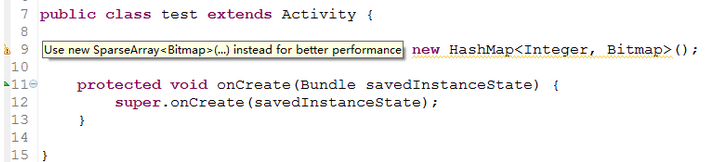# Android之SparseArray详解

最近编程时，发现一个针对HashMap<Integer, E>的一个提示：public SparseArray() {
this(10);
}

public SparseArray(int initialCapacity) {
......
}


public void put(int key, E value)
public void append(int key, E value)



	private static int binarySearch(int[] a, int start, int len, int key) {
int high = start + len;
int low = start - 1;

while (high - low > 1) {
int guess = (high + low) / 2;

if (a[guess] < key) {
low = guess;
continue;
}
high = guess;
}

if (high == start + len)
return start + len ^ 0xFFFFFFFF;
if (a[high] == key) {
return high;
}
return high ^ 0xFFFFFFFF;
}

public E get(int key)
public E get(int key, E valueIfKeyNotFound)

public int keyAt(int index)


public E valueAt(int index)

查看键所在位置，由于采用二分法查找键的位置，所以没有的话返回小于0的数值，而不是返回-1，这点要注意，返回的负数其实是表示它在哪个位置就找不到了，如果你存了5个，查找的键大于5个值的话，返回就是-6：

public int indexOfKey(int key)

public int indexOfValue(E value)

public void delete(int key)
public void remove(int key)

public void remove(int key) {
delete(key);
}
public void removeAt(int index)
public void clear()


public void setValueAt(int index, E value)
public void put(int key, E value)
put方法还可以修改键值对，注意：如果键不存在，就会变为添加新键值对

SparseArray实现了Cloneable接口，还可以调用clone方法。